# Method for drawing ray diagrams – convex lens

A convex lens ray diagram is a simple way of visualising the path that light rays take when passing through a convex lens.

To draw a ray diagram and find the location of the image that would be created on a screen you only need to draw two ray lines. This is sometimes referred to as "The two rules of refraction for converging lenses". The only thing to remember is:

From the object
1. Pass a parallel line through the principal focal point
2. Pass a ray line through the centre of the convex lens

## Here is each stage

1. From the object, pass a parallel line through the principal focal point. We usually choose the top of the object: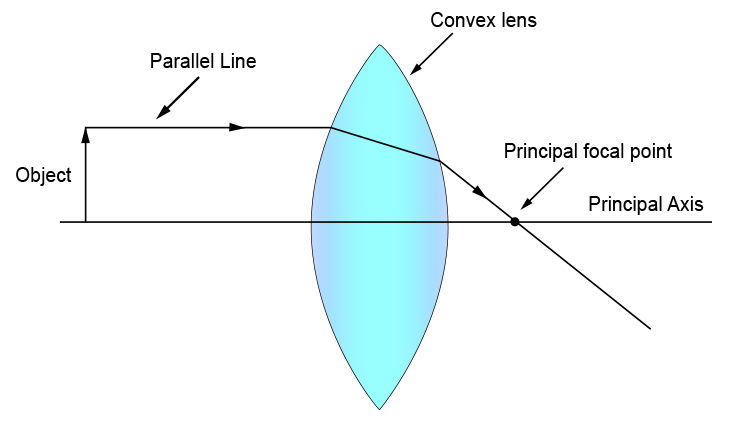2. From the object, pass a ray line through the centre of the convex lens.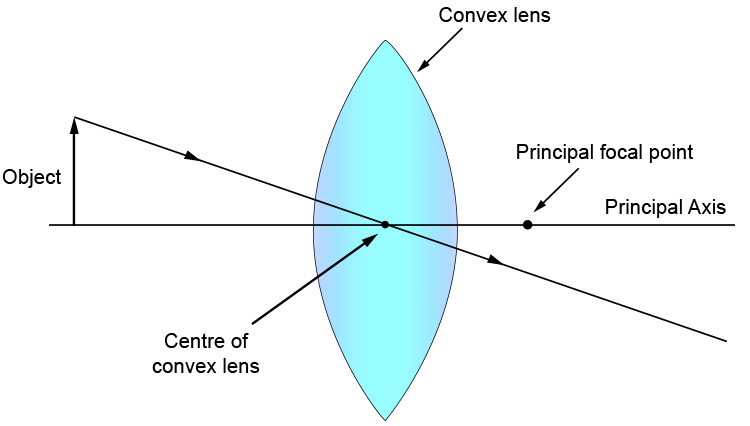Where these two lines intersect is the top of the image. Draw a line vertically up to the principal axis and you have your complete image as below: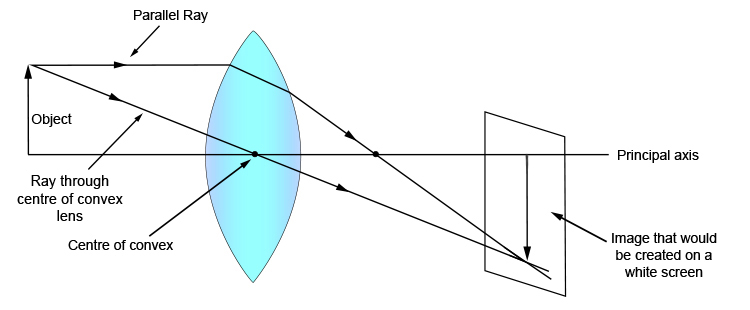And that is it. It's that easy.

We could finish the lesson there but Mammoth Memory just want to show you that you could in fact apply the same idea at any point on the object and the same ray diagram would work.

Let's try a ray diagram from the middle of the object.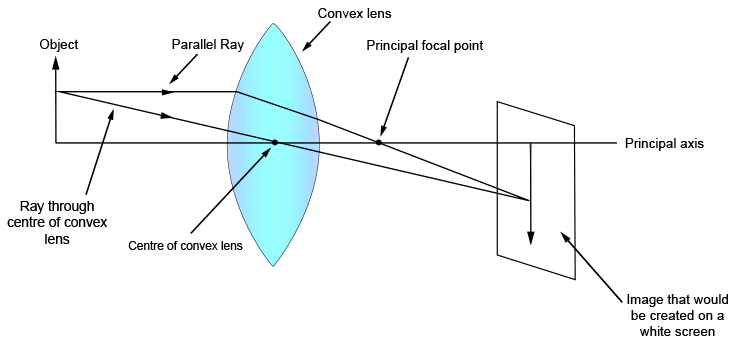You can see we could carry out this ray diagram method anywhere on the object, but again we emphasise that this is normally carried out from the top of the object.

NOTE: Some books inform you that there is one more rule for drawing ray diagrams and that is: from the object draw a line so that it passes through the object's side's principal focal point on the way to the lens. This ray line will then refract and will travel parallel to the principal axis.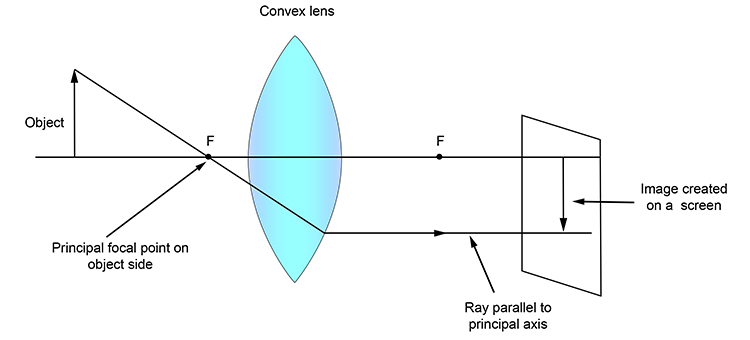The above is useful to know but not necessary in order to work out the image position. Only the first two rules are needed.